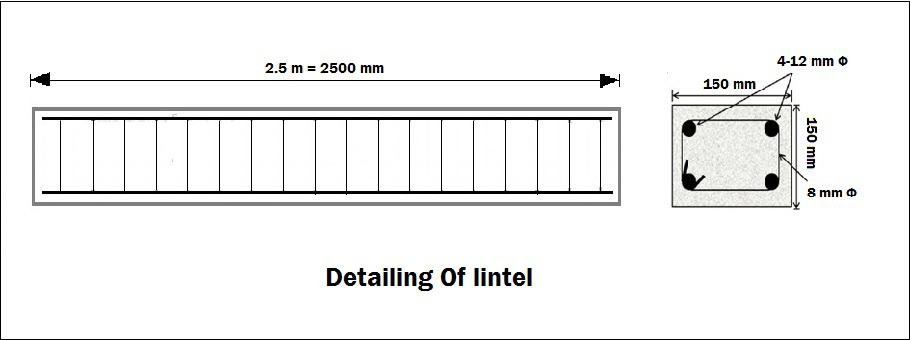# BBS Of Lintel Beam – Bar Bending Schedule Of Lintel Beam

## Bar Bending Schedule Of Lintel Beam:

In this article, I will discuss how to prepare BBS of RCC Lintel Beam.1. Calculate Total Length Of Main Bars:

Length of 1 bar = Length of lintel – clear cover for both sides

= 2500 – 2 x 25 [Clear cover for both sides]

=2450 mm

= 2.4 m.

Length of 4 bars = 2.4 x 4 = 9.6 m

2. Calculate Weight Of Steel For Main Bars:

Weight of steel for 12 mm bar = D²L/162 = 12² x 9.6/162 = 8.53 kg.

3. Calculate No Of Stirrups:

No of stirrups = (Total length of lintel/c/c distance between strriups) + 1

= (2500/150) + 1 = 18

4. Calculate Total Length Of Stirrups:

Inner depth distance = 150 -25 -25 -8 =84 mm

Width distance = 150 – 25 – 25 -8 = 84 mm.

Cutting length of stirrups =(2x Inner deoth diatance) +(2xWidth depth) + Hooks Length – Bend

Hooks length = 10d

Bend = 2d

We have 2 hooks and 5 bend

So,

Cutting length of stirrups = (2×84) + (2 x84) +2x10x8 -5x2x8 = 418 mm = 0.418 m

Total length of stirrups = 0.418 x 18 = 7.54 m

5. Calculate Weight Of Steel For Stirrups:

Weight of steel for stirrups = D²L/162 = 8² x 7.54/162 = 7.61 kg.

Total weight of steel for lintel = 8.53 + 7.61 = 16.14 kg.

### 2 thoughts on “BBS Of Lintel Beam – Bar Bending Schedule Of Lintel Beam”

1. Unit weight of steels as per NBC